Next: Spin Projection Operators Up: Projection Operators for Energy Previous: Projection Operators for Energy

## Energy Projection Operators

The positive and negative-energy projection operators can be guessed from the Dirac equation in momentum space, equation 5.187,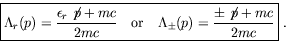(5.206)

Applying the trial'' positive-energy projection operator to an arbitrary Dirac spinor and using equation 5.187, we have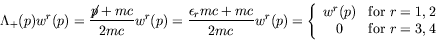(5.207)

and similarly for the negative-energy operator, as required.

Applying the operator twice we have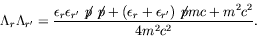(5.208)

Since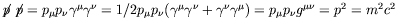,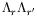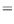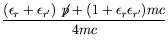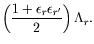(5.209)

Therefore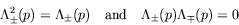(5.210)

as required of a projection operator.

Also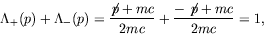(5.211)

as required.Next: Spin Projection Operators Up: Projection Operators for Energy Previous: Projection Operators for Energy
Douglas M. Gingrich (gingrich@ ualberta.ca)
2004-03-18